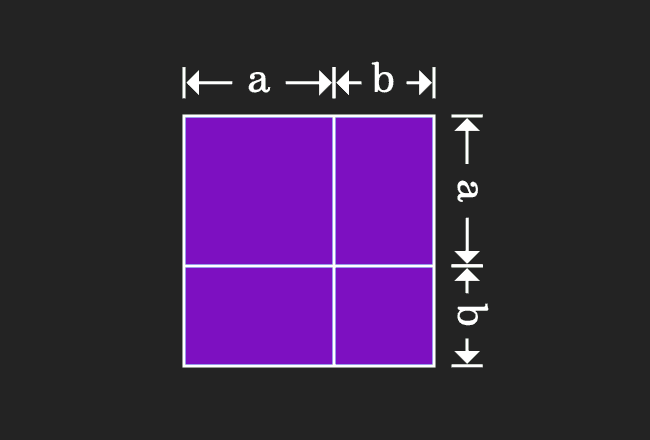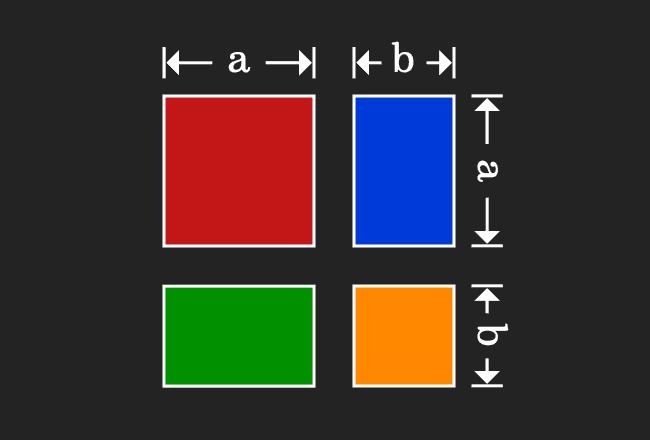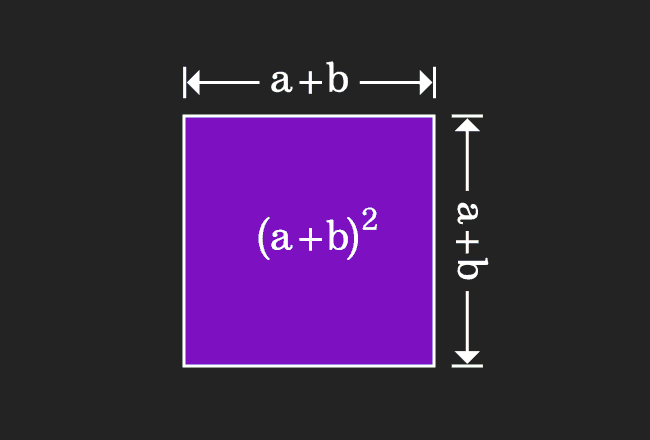# Proof of $(a+b)^2$ formula in Geometrical Method

The expansion of the algebraic identity a plus b whole square can be derived in mathematical form by the geometrical approach. In this method, the concept of the areas of the geometrical shapes squares and rectangles are used in proving the a plus b whole square formula.

### Calculate the Area of a Square

In this step, we evaluate the area of a square in algebraic form.1. Take a square and divide it into two different parts vertically. The lengths of them are $a$ and $b$ respectively.
2. Similarly, divide the square horizontally with same lengths. So, the widths of them are also $a$ and $b$ respectively.
3. The length and width of the square are same and the length of its each side is $a+b$. Therefore, the area of the square is $(a+b) \times (a+b)$ $\,=\,$ $(a+b)^2$ geometrically.

### Find the Areas of Geometric shapes

This geometrical procedure splits a square as four geometric shapes, in which two of them are different squares and remaining two are two rectangles. Now, evaluate the area of each geometrical shape in mathematical form.1. The length of each side of first square is $a$. So, its area is equal to $a^2$.
2. The dimension of one rectangle are $b$ and $a$. So, its area is equal to $ba$.
3. The dimension of second rectangle are $a$ and $b$. So, its area is equal to $ab$.
4. The length of each side of second square is $b$. So, its area is equal to $b^2$.

Now, add areas of the all four geometrical shapes to express the whole area in mathematical form.$a^2+ba+ab+b^2$

According to the commutative property of multiplication, the product of $a$ and $b$ is equal to the product of $b$ and $a$. The equality of the areas of both rectangles can also be proved geometrically.

Therefore, the term $ba$ can be written as $ab$ and vice-versa.

$\implies$ $a^2+ab+ab+b^2$

$\implies$ $a^2+2ab+b^2$

$\implies$ $a^2+b^2+2ab$

### Equality of the Areas of shapes

We are going to learn the equality property in the mathematical form.1. We have derived that the area of a square is equal to $(a+b)^2$ in the first step.
2. In the second step, it is proved that the sum of the areas of four geometric shapes is equal to $a^2+b^2+2ab$.

Actually, a square is divided as four geometrical shapes. It is obvious that the area of the square is equal to sum of the areas of them.

$\,\,\, \therefore \,\,\,\,\,\,$ ${(a+b)}^2$ $\,=\,$ $a^2 + b^2 + 2ab$

Geometrically, it is proved that square of $a+b$ can be expanded as $a$ squared plus $b$ squared plus two times product of $a$ and $b$.

Latest Math Topics
Jun 26, 2023
Jun 23, 2023

###### Math Questions

The math problems with solutions to learn how to solve a problem.

Learn solutions

Practice now

###### Math Videos

The math videos tutorials with visual graphics to learn every concept.

Watch now

###### Subscribe us

Get the latest math updates from the Math Doubts by subscribing us.